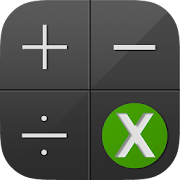# EquaCalc Scientific CalculatorEveryone
185
This app is scientific calculator with the ability to edit your printed data . Here is also list of fundamental physical constants

This application also can solve linear equations, quadratic equations cubic equations and systems of equations: a system of two, three and four equations. The complex numbers is supported in a solution of quadratic equations and cubic equations.

This application supports a matrix determinant and a matrix inverse.

Here is a reference: integrals, derivative of function, trigonometry, periodic table.

Soon in this app will be graphics, integrals and matrices support.

This application does not rely on an internet connection to solve equations and can function offline.
Collapse

Review Policy
4.5
185 total
5
4
3
2
1

## What's New

-Improvements
Collapse

Updated
November 15, 2015
Size
5.4M
Installs
5,000+
Current Version
2.1.0
Requires Android
4.1 and up
Content Rating
Everyone
Permissions
Offered By
Igor Erkin
Developer
РФ, г. Москва, ул. Москворечье